• 最近看论文，发现一个很不错的概率密度估计方法。在此小记一下。 先来看看准备知识。 密度估计经常在统计学中作为一种使用有限的样本来估计其概率密度函数的方法。 我们在研究随机变量的过程中，随机变量的概率...
最近看论文，发现一个很不错的概率密度估计方法。在此小记一下。
先来看看准备知识。
密度估计经常在统计学中作为一种使用有限的样本来估计其概率密度函数的方法。
我们在研究随机变量的过程中，随机变量的概率密度函数的作用是描述随机变量的特性。（概率密度函数是用来描述连续型随机变量取值的密集程度的，举例：某地某次考试的成绩近似服从均值为80的正态分布,即平均分是80分,由正态分布的图形知x=80时的函数值最大,即随机变量在80附近取值最密集,也即考试成绩在80分左右的人最多。）但是在实际应用中，总体概率密度函数通常是未知的，那么如何来估计总体概率密度呢？一般，我们通过抽样或者采集一定的样本，可以根据统计学知识从样本集合中推断总体概率密度。这种方法统称为概率密度估计，即根据训练样本来确定随机变量的概率分布。一般概率密度估计方法方法大致分为两类：参数估计和非参数估计。
参数估计(Parametric Estimation)是根据对问题的经验知识，假设问题具有某种数学模型 ，随机变量服从某种分布，即先假定概率密度函数的形式，然后通过训练数据估计出分布函数的参数。常见的参数估计方法有极大似然估计方法和贝叶斯估计方法。对于参数估计，根据样本中是否已知样本所属类别（即是否带标签）将参数估计又划分为监督参数估计和非监督参数估计。监督参数估计是由 已知类别的样本集对总体分布的某些参数进行统计推断 。而无监督参数估计已知总体概率密度函数形式但未知样本所属的类别，要求推断出概率密度函数的某些参数 ，这种推断方法称之为非监督情况下的参数估计。
非参数估计(Nonparametric Estimation)是在已知样本所属的类别不假定总体分布形式下， 基于大样本的性质，直接利用样本估计出整个函数。在很多情况下，我们对样本的分布并没有充分的了解，无法事先给出密度函数的形式，而且有些样本分布的情况也很难用简单的函数来描述。在这种情况下，就需要用到非参数估计。但是，并不是非参数估计一定优于参数估计，因为非参数估计受训练样本影响，其完备性或泛化能力不会很好；且这种估计只能用数值方法取得，无法得到完美的封闭函数图形。常用的非参数估计方法有直方图法，核概率密度估计等。
（若有闲暇，后续将极大似然、贝叶斯估计等等估计方法详细总结）
这里说说核密度估计方法（也有称之为Parzen$Parzen$窗法）
进入正题
核密度估计的形式：
f^h(x)=1n∑i=1nKh(x−xi)=1nh∑i=1nK(x−xih)\hat{f}_h(x)=\frac{1}{n}\sum_{i=1}^nK_h(x-x_i)=\frac{1}{nh}\sum_{i=1}^nK\left( \frac{x-x_i}{h} \right)
这里K(x)$K(x)$ 是核函数（非负，积分为1，均值为0，符合概率密度的性质），h>0$h>0$是带宽。有很多种核函数，uniform,triangular,biweight,triweight,Epanechnikov,normal$uniform,triangular, biweight, triweight, Epanechnikov,normal$等。各种核函数的图形如下：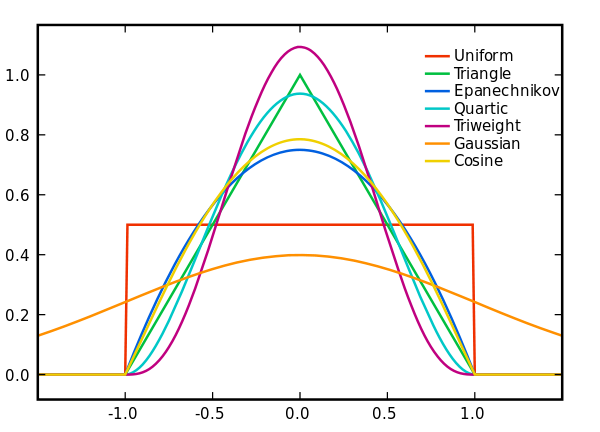由于高斯内核方便的数学性质，也经常使用 K(x)=ϕ(x)$K(x)= ϕ(x)$，ϕ(x)$ϕ(x)$为标准正态概率密度函数。核密度估计与直方图很类似，但相比于直方图还有光滑连续的性质。
举例理解（该例子来自维基百科https://en.wikipedia.org/wiki/Kernel_density_estimation）
已知：6个数据点
x1=−2.1，x2=−1.3，x3=−0.4，x4=1.9，x5=5.1，x6=6.2$x_1 = -2.1，x_2 = -1.3，x_3 = -0.4，x_4 = 1.9，x_5 = 5.1，x_6 = 6.2$
对于直方图，首先将水平轴划分为覆盖数据范围的子间隔或区段。在这种情况下，我们有6个宽度为2的矩形。每当数据点落在此间隔内时，我们放置一个高度为112$\frac{1}{12}$的矩形。对于核密度估计，我们在每个数据点xi$x_i$上放置方差2.25（由红色虚线表示）的正态核函数。叠加一起得到核密度估计的结果，蓝色线表示。
很明显，直方图得到的密度估计平滑程度比使用核密度估计得到的密度函数要差很多.
现在问题是如何选定核函数的“方差”呢？这其实是由h$h$来决定，不同的带宽h$h$下的核函数估计结果差异很大。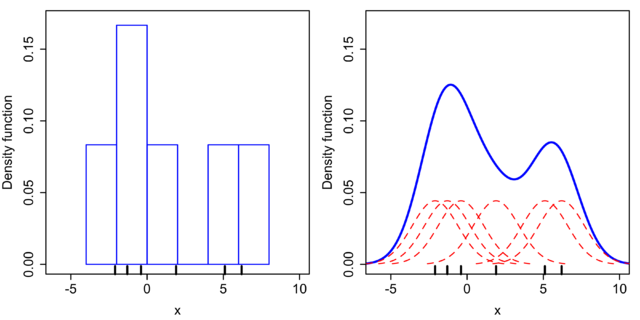核带宽的选择
带宽是一个自由参数，对所得到的估计值有很大的影响。为了说明效果，举个例子：
下图是从标准正态分布中抽取的随机样本（横轴上的蓝色的点点代表样本点）灰色曲线是真是的概率密度（正态密度，均值0，方差1）。相比之下，红色曲线是使用了过小的带宽h(=0.05)$h(=0.05)$得出的概率密度曲线，可见其波折陡峭；绿色曲线过于平滑，因为它使用了过大的带宽h(=2)$h(=2)$，掩盖了数据大部分基础结构。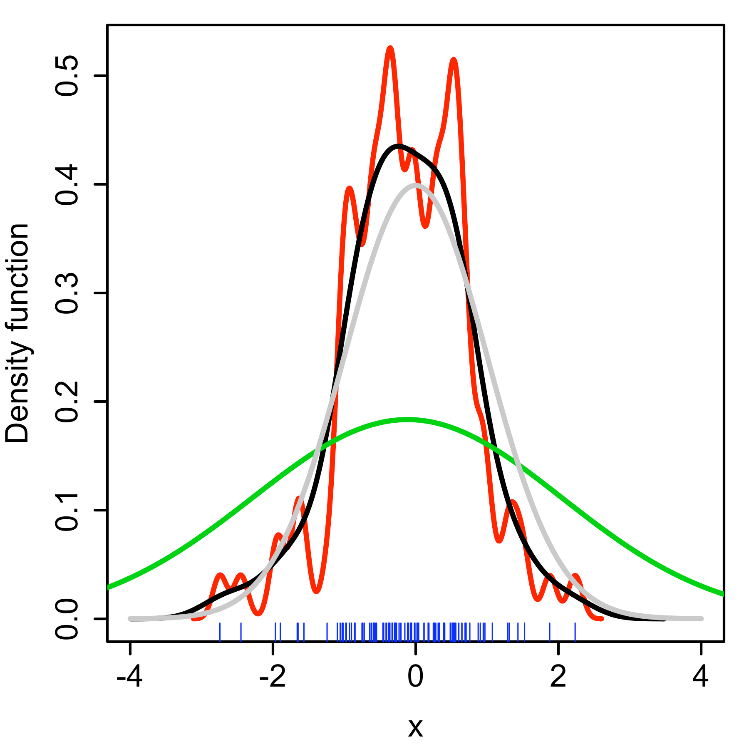那么对于h$h$的选择可以使用最小化L2风险函数（即平均积分平方误差,mean intergrated squared error）。
MISE(h)=E[∫(f^h(x)−f(x))2dx]MISE(h)=E\left[ \int (\hat{f}_h(x)-f(x))^2dx \right]

在weakassumptions$weak\, assumptions$下，MISE(h)=AMISE(h)+o(1nh+h4)$MISE (h) =AMISE(h) + o(\frac{1}{nh} + h^4)$，其中AMISE$AMISE$为渐进的MIS$MIS$E。而AMISE$AMISE$有，
AMISE(h)=R(K)nh+14m2(K)2h4R(f′′)AMISE(h)=\frac{R(K)}{nh} + \frac{1}{4}m_2(K)^2h^4R(f'')
其中，
R(g)=∫g(x)2dxR(g)=\int g(x)^2dx
m2(K)=∫x2K(x)dxm_2(K)=\int x^2K(x)dx
为了使MISE(h)$MISE(h)$最小，则转化为求极点问题，
∂AMISE(h)∂h=−R(K)nh2+m2(K)2h3R(f′′)=0\frac{\partial \, AMISE(h)}{\partial h} =-\frac{R(K)}{nh^2}+m_2(K)^2h^3R(f'')=0
hAMISE=R(K)15m2(K)25R(f′′)15n15h_{AMISE}=\frac{R(K)^{\frac{1}{5}}}{m_2(K)^{\frac{2}{5}}R(f'')^{\frac{1}{5}}n^{\frac{1}{5}}}
当核函数确定之后，hAMISE$h_{AMISE}$公式里的R$R$、m$m$、f′′$f''$都可以确定下来，有（hAMISE∼n−15$h_{AMISE}\sim n-\frac{1}{5}$），AMISE(h)=O(n−45)$AMISE(h) = O(n−\frac{4}{5})$。

如果使用高斯核函数进行核密度估计，则h$h$的最优选择（即使平均积分平方误差最小化的带宽）为
h=(4σ^53n)15≈1.06σ^n−15 h=\left (  \frac{4 \hat{\sigma}^5 }{3n} \right )^{\frac{1}{5}} \approx 1.06\hat{\sigma}n^{-\frac{1}{5}}
这里σ^$\hat{\sigma}$是样品的标准差。这种近似称为正态分布近似，高斯近似，或Silverman（1986）经验法则。虽然这个经验法则很容易计算，但应谨慎使用，因为当密度不接近正态时，可能会产生泛化极差的估计。该经验法则推导过程详见:Silverman, B.W. (1986). Density Estimation for Statistics and Data Analysis. London: Chapman & Hall/CRC. p. 48. ISBN 0-412-24620-1.
这里带宽的作用简述：
1.在数据可视化的相关领域中，带宽的大小决定了核密度估计函数（KDE）的平滑（smooth）程度，带宽越小越undersmooth，带宽越大越oversmooth。（详细解释）
2.在POI兴趣点推荐领域，或位置服务领域，带宽h$h$的设置主要与分析尺度以及地理现象特点有关。较小的带宽可以使密度分布结果中出现较多的高值或低值区域，适合于揭示密度分布的局部特征，而较大的带宽可以在全局尺度下使热点区域体现得更加明显。另外，带宽应与兴趣点的离散程度呈正相关，对于稀疏型的兴趣点分布应采用较大的带宽，而对于密集型的兴趣点则应考虑较小一些的带宽。

如果带宽不是固定的，而是根据样本的位置而变化(其变化取决于估计的位置（balloon  estimator）或样本点（逐点估计pointwise estimator）)，则会产生一种特别有力的方法，称为自适应或可变带宽的核密度估计。就POI兴趣点推荐来说，由于密集的城市地区的签到密度很高，人烟稀少的农村地区的签到密度较低。就是说不同位置应该采取不同的分析尺度，因此本文采用不固定的带宽来进行核密度估计。

说到这， 有些朋友可能不知道POI兴趣点推荐是啥意思， 这里简单的说一下：POI是Point-of-Interest的意思，即兴趣点。就是说，给用户推荐其感兴趣的地点。就这么简单。在推荐系统相关领域，兴趣点推荐是一个非常火爆的研究课题。这里会用到核密度估计的方法，比如这篇论文：Jia-Dong Zhang,Chi-Yin Chow.(2015)GeoSoCa: Exploiting Geographical, Social and Categorical Correlations for Point-of-Interest Recommendations.SIGIR’15, August 09 - 13, 2015, Santiago, Chile.就利用了可变带宽的核密度估计方法。

这里再简单讨论一下自适应带宽的核密度估计方法。自适应带宽的核密度估计方法是在固定带宽核密度函数的基础上，通过修正带宽参数为而得到的，其形式如式所示：
k(x)=1M∑j−1M1(ωhj)nK(x−x(j)ωhj)k(x)=\frac{1}{M}\sum_{j-1}^M \frac{1}{(\omega h_j)^n}K\left( \frac{x-x^{(j)}}{\omega h_j} \right)
K(x)=1(2π)n|S−−−−−−√|exp(−12xTS−1x)K(x)=\frac{1}{\sqrt{(2\pi)^n|S}|}\exp\left( -\frac{1}{2}x^TS^{-1}x \right)
hj=⎧⎩⎨⎪⎪⎪⎪⎪⎪[∏Mk=1f(x(k))]1Mf(x(j))⎫⎭⎬⎪⎪⎪⎪⎪⎪αh_j=\left \{ \frac{\left [ \prod_{k=1}^{M}f(x^{(k)}) \right ]^{\frac{1}{M}}}{f(x^{(j)})}\right \}^\alpha

这里k(x)$k(x)$是带宽为hj$h_j$的核密度估计函数，M$M$是样例的个数，看出来了吧，每一个点j$j$都有一个带宽hj$h_j$，因此这叫自适应或可变。K(x)$K(x)$是核函数，这里用了高斯核函数，当然也可以是其他的核函数。0≤α≤1$0 \leq \alpha \leq 1$，为灵敏因子，通常α$\alpha$取0.5，α=0$\alpha=0$时，自适应带宽的核密度估计就变成了固定带宽的核密度估计了。固定带宽的核密度估计就是前面说的核密度估计。ω$\omega$表示带宽的参数。
自适应带宽的核密度估计可以参考维基百科：https://en.wikipedia.org/wiki/Variable_kernel_density_estimation

推荐帖子：http://blog.sina.com.cn/s/blog_62b37bfe0101homb.html

参考资料
https://en.wikipedia.org/wiki/Kernel_density_estimation
https://en.wikipedia.org/wiki/Variable_kernel_density_estimation
http://www.tuicool.com/articles/EVJnI3
袁修开,吕震宙,池巧君. 基于核密度估计的自适应重要抽样可靠性灵敏度分析.西北工业大学学报.Vol.26 No.3.2008.6.
展开全文统计学 非参数估计
• 用matlab实现对6个样本点实现直方图估计、高斯核密度估计
• ## 非参数估计：核密度估计KDE

万次阅读 多人点赞 2016-12-14 11:38:46
http://blog.csdn.net/pipisorry/article/details/53635895核密度估计Kernel Density Estimation(KDE)概述密度估计的问题由给定样本集合求解随机变量的分布密度函数问题是概率统计学的基本问题之一。解决这一问题的...
http://blog.csdn.net/pipisorry/article/details/53635895核密度估计Kernel Density Estimation(KDE)概述密度估计的问题由给定样本集合求解随机变量的分布密度函数问题是概率统计学的基本问题之一。解决这一问题的方法包括参数估计和非参数估计。参数估计参数估计又可分为参数回归分析和参数判别分析。在参数回归分析中，人们假定数据分布符合某种特定的性态，如线性、可化线性或指数性态等，然后在目标函数族中寻找特定的解，即确定回归模型中的未知参数。在参数判别分析中，人们需要假定作为判别依据的、随机取值的数据样本在各个可能的类别中都服从特定的分布。经验和理论说明，参数模型的这种基本假定与实际的物理模型之间常常存在较大的差距，这些方法并非总能取得令人满意的结果。[参数估计：最大似然估计MLE][参数估计：文本分析的参数估计方法]非参数估计方法由于上述缺陷，Rosenblatt和Parzen提出了非参数估计方法，即核密度估计方法。由于核密度估计方法不利用有关数据分布的先验知识，对数据分布不附加任何假定，是一种从数据样本本身出发研究数据分布特征的方法，因而，在统计学理论和应用领域均受到高度的重视。核密度估计（kernel density estimation）是在概率论中用来估计未知的密度函数，属于非参数检验方法之一，由Rosenblatt (1955)和Emanuel Parzen(1962)提出，又名Parzen窗（Parzen window）。Ruppert和Cline基于数据集密度函数聚类算法提出修订的核密度估计方法。核密度估计在估计边界区域的时候会出现边界效应。[https://zh.wikipedia.org/zh-hans/核密度估计]因此，一句话概括，核密度估计Kernel Density Estimation(KDE)是在概率论中用来估计未知的密度函数，属于非参数检验方法之一。在密度函数估计中有一种方法是被广泛应用的——直方图。如下图中的第一和第二幅图（名为Histogram和Histogram, bins shifted）。直方图的特点是简单易懂，但缺点在于以下三个方面：密度函数是不平滑的；密度函数受子区间（即每个直方体）宽度影响很大，同样的原始数据如果取不同的子区间范围，那么展示的结果可能是完全不同的。如下图中的前两个图，第二个图只是在第一个图的基础上，划分区间增加了0.75，但展现出的密度函数却看起来差异很大；直方图最多只能展示2维数据，如果维度更多则无法有效展示。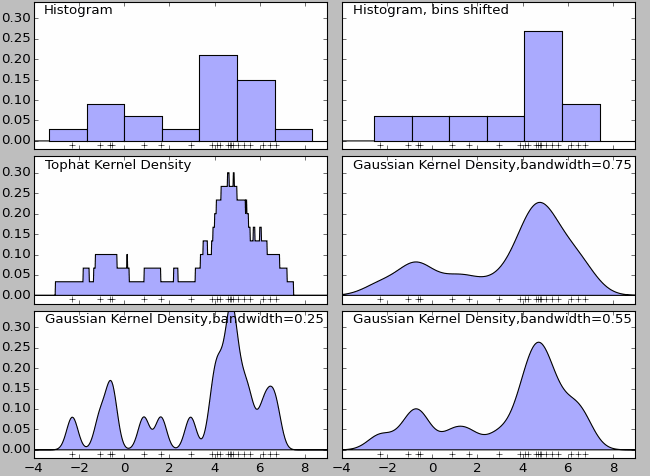核密度估计有多种内核，图3（Tophat Kernl Density）为不平滑内核，图4（Gaussian Kernel Density,bandwidth=0.75）为平滑内核。在很多情况下，平滑内核（如高斯核密度估计，Gaussian Kernel Density）使用场景较多。虽然采用不同的核函数都可以获得一致性的结论（整体趋势和密度分布规律性基本一致），但核密度函数也不是完美的。除了核算法的选择外，带宽（bandwidth）也会影响密度估计，过大或过小的带宽值都会影响估计结果。如上图中的最后三个图，名为Gaussian Kernel Density,bandwidth=0.75、Gaussian Kernel Density,bandwidth=0.25、Gaussian Kernel Density,bandwidth=0.55.核密度估计的应用场景股票、金融等风险预测：在单变量核密度估计的基础上，可以建立风险价值的预测模型。通过对核密度估计变异系数的加权处理，可以建立不同的风险价值的预测模型。密度估计中应用较多的算法是高斯混合模型以及基于近邻的核密度估计。高斯混合核密度估计模型更多会在聚类场景中应用。[核密度估计Kernel Density Estimation(KDE)]核密度分析可用于测量建筑密度、获取犯罪情况报告，以及发现对城镇或野生动物栖息地造成影响的道路或公共设施管线。可使用 population 字段根据要素的重要程度赋予某些要素比其他要素更大的权重，该字段还允许使用一个点表示多个观察对象。例如，一个地址可以表示一栋六单元的公寓，或者在确定总体犯罪率时可赋予某些罪行比其他罪行更大的权重。对于线要素，分车道高速公路可能比狭窄的土路产生更大的影响，高压线要比标准电线杆产生更大的影响。[ArcGIS中的介绍]热力图大家一定听说过，其实热力图就是核密度估计。总而言之，核密度就是用来估计密度的，如果你有一系列空间点数据，那么核密度估计往往是比较好的可视化方法皮皮blog核密度估计所谓核密度估计，就是采用平滑的峰值函数(“核”)来拟合观察到的数据点，从而对真实的概率分布曲线进行模拟。核密度估计（Kernel density estimation），是一种用于估计概率密度函数的非参数方法，为独立同分布F的n个样本点，设其概率密度函数为f，核密度估计为以下：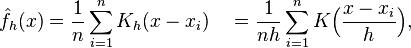K(.)为核函数（非负、积分为1，符合概率密度性质，并且均值为0）。有很多种核函数，uniform,triangular, biweight, triweight, Epanechnikov,normal等。h>0为一个平滑参数，称作带宽(bandwidth)，也看到有人叫窗口。Kh(x) = 1/h K(x/h). 为缩放核函数(scaled Kernel)。核密度函数的原理比较简单，在我们知道某一事物的概率分布的情况下，如果某一个数在观察中出现了，我们可以认为这个数的概率密度很大，和这个数比较近的数的概率密度也会比较大，而那些离这个数远的数的概率密度会比较小。基于这种想法，针对观察中的第一个数，我们可以用K去拟合我们想象中的那个远小近大概率密度。对每一个观察数拟合出的多个概率密度分布函数，取平均。如果某些数是比较重要的，则可以取加权平均。需要说明的一点是，核密度的估计并不是找到真正的分布函数。Note: 核密度估计其实就是通过核函数（如高斯）将每个数据点的数据+带宽当作核函数的参数，得到N个核函数，再线性叠加就形成了核密度的估计函数，归一化后就是核密度概率密度函数了。以下面3个数据点的一维数据集为例：5, 10, 15绘制成直方图是这样的：                                                   而使用KDE则是：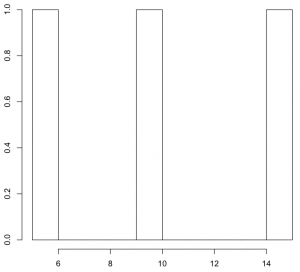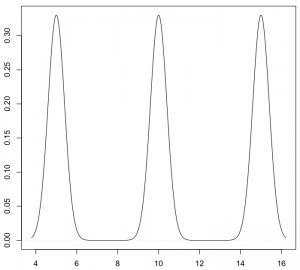KDE核函数k(.)理论上，所有平滑的峰值函数均可作为KDE的核函数来使用，只要对归一化后的KDE而言(描绘在图上的是数据点出现的概率值)，该函数曲线下方的面积和等于1即可。 只有一个数据点时，单个波峰下方的面积为1，存在多个数据点时，所有波峰下方的面积之和为1。概而言之，函数曲线需囊括所有可能出现的数据值的情况。常用的核函数有：矩形、Epanechnikov曲线、高斯曲线等。这些函数存在共同的特点：在数据点处为波峰；曲线下方面积为1。单个数据点（只有一个数据时）所对应的这些核函数矩形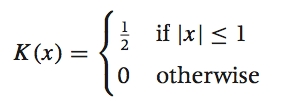Epanechnikov曲线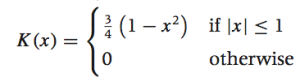高斯曲线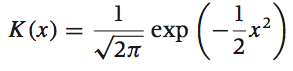[概率论：高斯/正态分布                    ]sklearn中实现的核函数sklearn核函数形式Gaussian kernel (kernel = 'gaussian')Tophat kernel (kernel = 'tophat')ifEpanechnikov kernel (kernel = 'epanechnikov')Exponential kernel (kernel = 'exponential')Linear kernel (kernel = 'linear')ifCosine kernel (kernel = 'cosine')if[Kernel Density Estimation¶]wekipedia上各种核函数的图形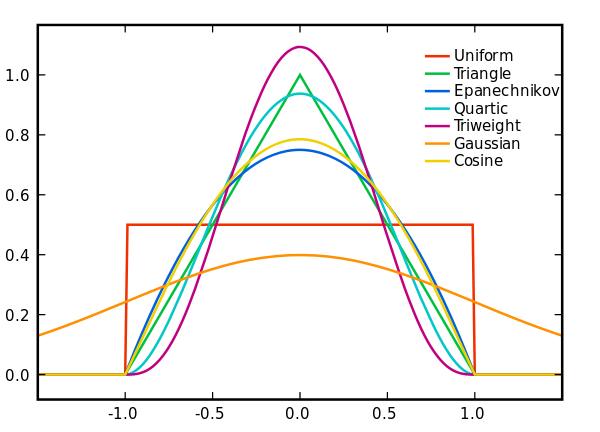均匀核函数 k(x)=1/2,-1≤x≤1 加入带宽h后： kh(x)=1/(2h),-h≤x≤h三角核函数 k(x)=1-|x|,-1≤x≤1 加入带宽h后： kh(x)=(h-|x|)/h^2,-h≤x≤h伽马核函数 kxi(x)=[x^(α-1)exp{-xα/xi}]/[(xi/α)^α.Γ(α)]高斯核函数K(x,xc)=exp(-||x-xc||^2/(2*σ)^2)其中xc为核函数中心,σ为函数的宽度参数[https://zh.wikipedia.org/zh-hans/%E6%A0%B8%E5%AF%86%E5%BA%A6%E4%BC%B0%E8%AE%A1]不同内核的比较 Epanechnikov 内核在均方误差意义下是最优的，效率损失也很小。由于高斯内核方便的数学性质，也经常使用 K(x)= ϕ(x)，ϕ(x)为标准正态概率密度函数。对于多个数据点的KDE曲线：由于相邻波峰之间会发生波形合成，因此最终所形成的曲线形状与选择的核函数关系并不密切。考虑到函数在波形合成计算上的易用性，一般使用高斯曲线(正态分布曲线)作为KDE的核函数。KDE算法：索引树lz发现sklearn算法实现中有一个参数是算法项，如algorithm='auto'，想了一下是为了加速。KDE的概率密度函数公式得到后有了上述公式之后，只需遍历输出图像的每一个点，计算其核密度估计值即可。但是稍微想一下就发现这个程序太冗余了，如果有很多点（n很大），并且输出图像很大，那么每一个像素都需要进行n个累积的加法运算，并且大部分都是+0（因为一般来说，一个点附近的点不会很多，远远小于n，其余大部分点与这个像素的距离都大于r），这样就造成了冗余计算。解决方案当然也非常简单，就是建立一个索引，然后在计算某个像素的核密度估计值时利用索引搜索出附近的点，然后累积这些点的核函数即可。如Dotspatial自带了多种空间索引，有R树，R*树，KD树等；sklearn自带了kd tree, ball tree等等。如果只需找出附近的点，对索引要求不高，任意一个索引都能使用。[空间点云核密度估计算法的实现-以Dotspatial为基础GIS库]KDE带宽h如何选定核函数的“方差”呢？这其实是由带宽h来决定，不同的带宽下的核函数估计结果差异很大。带宽反映了KDE曲线整体的平坦程度，也即观察到的数据点在KDE曲线形成过程中所占的比重。带宽越大，观察到的数据点在最终形成的曲线形状中所占比重越小，KDE整体曲线就越平坦；带宽越小，观察到的数据点在最终形成的曲线形状中所占比重越大，KDE整体曲线就越陡峭。还是以上面3个数据点的一维数据集为例，如果增加带宽，那么生成的KDE曲线就会变平坦：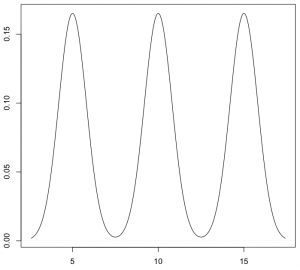如果进一步增加带宽，那么KDE曲线在变平坦的同时，还会发生波形合成：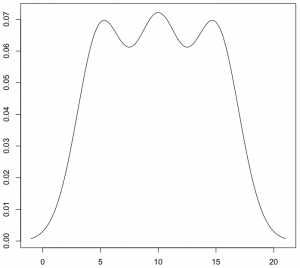相反，如果减少带宽，那么KDE曲线就会变得更加陡峭：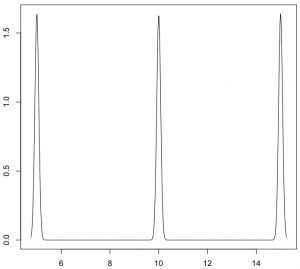从数学上来说，对于数据点Xi，如果带宽为h，那么在Xi处所形成的曲线函数为(其中K为核函数)：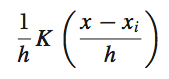在上面的函数中，K函数内部的h分母用于调整KDE曲线的宽幅，而K函数外部的h分母则用于保证曲线下方的面积符合KDE的规则(KDE曲线下方面积和为1)。带宽的选择带宽的选择很大程度上取决于主观判断：如果认为真实的概率分布曲线是比较平坦的，那么就选择较大的带宽；相反，如果认为真实的概率分布曲线是比较陡峭的，那么就选择较小的带宽。带宽计算好像也有相应的方法，如R语言中计算带宽时，默认采用”nrd0″方法。如何选择h？显然是选择可以使误差最小的。下面用平均积分平方误差（mean intergrated squared error）的大小来衡量h的优劣。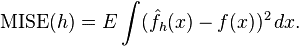在weak assumptions下，MISE (h) =AMISE(h) + o(1/(nh) + h4) ，其中AMISE为渐进的MISE。而AMISE有，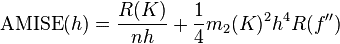，其中，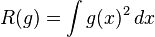，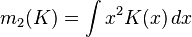为了使MISE(h)最小，则转化为求极点问题，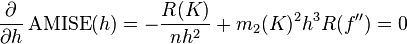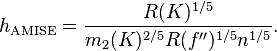当核函数确定之后，h公式里的R、m、f''都可以确定下来，有（hAMISE ~ n−1/5），AMISE(h) = O(n−4/5)。        如果带宽不是固定的，其变化取决于估计的位置（balloon  estimator）或样本点（逐点估计pointwise estimator），由此可以产产生一个非常强大的方法称为自适应或可变带宽核密度估计。[核密度估计(Kernel density estimation)                    ]在选择合适的核函数及带宽后，KDE可以模拟真实的概率分布曲线，并得到平滑而漂亮的结果。以近200个点的CPU使用率为例，使用KDE绘制的结果为：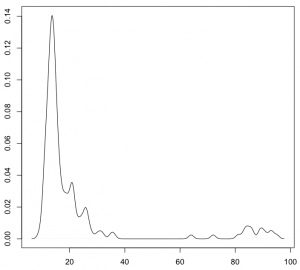[一维数据可视化：核密度估计(Kernel Density Estimates)]皮皮blog核密度估计的实现Python中KDE的实现：sklearn[sklearn.neighbors.KernelDensity(bandwidth=1.0, algorithm='auto', kernel='gaussian', metric='euclidean', atol=0, rtol=0, breadth_first=True, leaf_size=40, metric_params=None)from sklearn.neighbors import kde
import numpy as np

X = np.array([[-1, -1], [-2, -1], [-3, -2], [1, 1], [2, 1], [3, 2]])
kde = kde.KernelDensity(kernel='gaussian', bandwidth=0.2).fit(X)
print(kde.score_samples(X))
print(np.exp(kde.score_samples(X)))[-0.41075698 -0.41075698 -0.41076071 -0.41075698 -0.41075698 -0.41076071][ 0.66314807  0.66314807  0.6631456   0.66314807  0.66314807  0.6631456 ]score_samples(X)Evaluate the density model on the data.Parameters:X : array_like, shape (n_samples, n_features)kde.score_samples(X)返回的是点x对应概率的log值，要使用exp求指数还原。Note: 还原后的所有点的概率和范围是[0, 无穷大]，只是说一维数据线下面的面积或者二维数据面下面的体积和为1。[Density Estimation¶][sklearn.neighbors.KernelDensity¶]spark中KDE的实现MLlib中，仅仅支持以高斯核做核密度估计。[核密度估计]R中KDE的实现在R语言中，KDE的绘制是通过density()函数来实现的 — 通过density()函数计算得到KDE模型，然后再使用plot()函数对KDE曲线进行绘制：x <- c(5, 10, 15)plot(density(x))出于兼容性上的考虑，R语言中density()函数在计算带宽时，默认采用”nrd0″方法。不过，根据R语言的帮助文档，带宽参数bw应该显式声明为其它更合适的方法，比如”SJ”：plot(density(x, bw="SJ"))对于调整带宽，除了修改bw参数，还可以通过设定adjust参数来进行扩大或缩小：plot(density(x, bw="SJ", adjust=1.5))在上面的例子中，最终使用的带宽将是采用”SJ”方法计算得到的带宽的1.5倍。adjust参数的默认值为1，也即既不扩大、也不缩小。至于核函数，density()默认采用高斯曲线。可以通过设定kernel参数来更改核函数。比如：plot(density(x, bw="SJ", kernel="epanechnikov"))density()函数接受以下7个核函数选项：    gaussian。高斯曲线，默认选项。在数据点处模拟正态分布。    epanechnikov。Epanechnikov曲线。    rectangular。矩形核函数。    triangular。三角形核函数。    biweight。    cosine。余弦曲线。    optcosine。from: http://blog.csdn.net/pipisorry/article/details/53635895ref: [有边界区间上的核密度估计]
展开全文kde 非参数估计
• 目标检测，也叫目标提取，是一种基于目标几何和统计特征的图像分割，它将目标的分割和识别合二为一，其准确性和实时性是整个系统的一项重要能力。尤其是在复杂场景中，需要对多个目标进行实时处理时，目标自动提取和...
目标检测，也叫目标提取，是一种基于目标几何和统计特征的图像分割，它将目标的分割和识别合二为一，其准确性和实时性是整个系统的一项重要能力。尤其是在复杂场景中，需要对多个目标进行实时处理时，目标自动提取和识别就显得特别重要。

随着计算机技术的发展和计算机视觉原理的广泛应用，利用计算机图像处理技术对目标进行实时跟踪研究越来越热门，对目标进行动态实时跟踪定位在智能化交通系统、智能监控系统、军事目标检测及医学导航手术中手术器械定位等方面具有广泛的应用价值。

本文介绍高斯核密度估计和Ep核密度估计两种核模型算法：

1、Epanechnikov核函数介绍

核密度估计是在概率论中用来估计未知的密度函数，属于非参数检验方法之一，由Rosenblatt (1955)和Emanuel Parzen(1962)提出，又名Parzen窗（Parzen window）。

假设我们有n个数X1-Xn,我们要计算某一个数X的概率密度有多大。核密度估计的方法是这样的：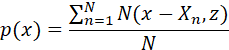(1)

其中N(x,z)为正态分布的概率密度函数,z为设定的参数。

核密度估计算法的基本原理是在对某一事物的概率分布的情况下，假设一个数在观察的过程中出现了，就可以假定这个数相对应的概率密度比较大，从而可以得出和这个数相邻的数的概率密度也会比较大，反之，离这个数较远的数的概率密度比较小。基于这一原理，我们可以通过一个函数来映射出每个数概率密度之间的关系。

假设xi,i=1,2,…N为同一未知概率密度函数产生的维度为d的N个样本点。核密度估计函数表示如下：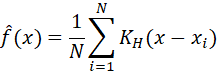(2)

其中KH为核函数，可以表示为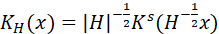。H为d×d大小的正定带宽矩阵。当简化带宽矩阵为单参数h时，(2)式变为我们所熟悉的形式：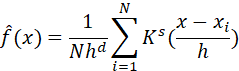(3)

多维变量的核函数Ks可以通过每个维度下单变量核函数Kj的乘积计算来表示：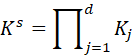(4)

xi∈{x1,x2,…,xN}为图像中位于坐标(x0,y0)的像素点在第i时刻的色彩向量，{x1,x2,…,xN}为当前像素样本集Sa。对于t时刻此像素点的观测值xt而言，其密度函数p(xt)的核密度估计则可定义如下：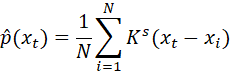(5)

其中，Ks为选择的核函数，满足性质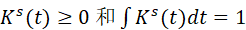。

所采集的图像数据一般为多通道的彩色图像(例如RGB三通道)，利用公式(2)、(3)，可以写出d维颜色通道的核函数乘积：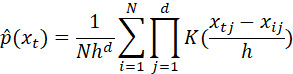(6)

单维度Epanechnikov函数表达式如下：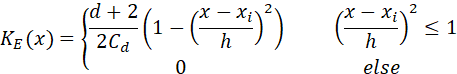(7)

Cd表示d维超球体体积，以RGB图像为例，C3是半径为1的球的体积，即C3=4π∙13/3。此时像素的输入xt=xt1,xt2,xt3，样本点为xi=[xi1,xi2,xi3]。基于Epanechnikov核函数的三通道彩色图像像素值的概率密度估计函数为：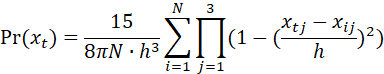(8)

2、高斯核函数介绍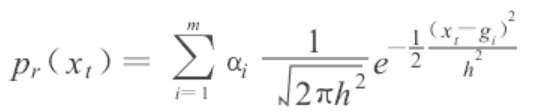展开全文• 核密度估计属于非参数估计，它主要解决的问题就是在对总体样本的分布未知的情况，如何估计样本的概率分布。 像平时，我们经常也会用直方图来展示样本数据的分布情况，如下图： 但是，直方图有着明显的缺点： 非常...
核密度估计属于非参数估计，它主要解决的问题就是在对总体样本的分布未知的情况，如何估计样本的概率分布。
像平时，我们经常也会用直方图来展示样本数据的分布情况，如下图：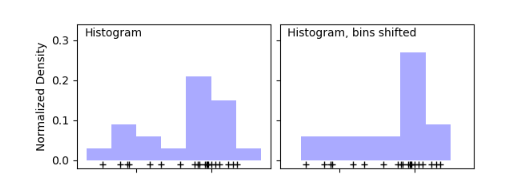但是，直方图有着明显的缺点：

非常不平滑，邻近的数据无法体现它们的差别；
不同的bins画出的直方图差别非常大；
无法计算概率密度值。

核密度估计
核密度估计就可以很好的解决直方图存在的问题，它的原理其实也很简单：当你需要估计一个点的概率密度值的时候，根据待估计点与每一个样本点的距离计算出一个密度值，距离越近，得到的密度值就越大，越远的话就越小，最后将所有密度值加权平均就得到该估计点在样本分布中的一个概率密度值了。
那为什么是这个原理呢？其实也很好理解，比如我们的该估计点处在样本点很密集的位置，不用算我们就自然就认为它的概率密度值也比较大。此时有许多样本点离待估计点很近，上面也说到离样本点的距离越近得到密度值就越大，那么也就意味着用于加权平均的密度值中有许多数值较大，算出来的最终密度值自然也会比较大。
具体公式如下：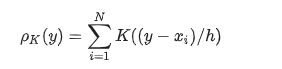y是待估计点，xi就是样本点，i=1,2,…,N
K(x;h)是带有参数h的核函数，这里h的作用决定了核函数估计出来的分布的平滑程度，h越大，分布就会越平滑。
多维数据的核密度估计
对于多维数据，在计算密度值的时候，需要对每个维度的密度值进行累乘，具体公式如下：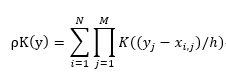M就是数据的维度，y(j)是待估计点的第j维，x(i,j)是第i个样本点的第j维
但是，如果我们在对估计一个点的概率密度值的时候，如果将所有样本点都考虑进来计算的话，会非常的冗余。其实，我们可以就只考虑离待估计点比较相近的样本点，因为离得比较远的样本点，对该估计点的密度值贡献也非常小，甚至许多为0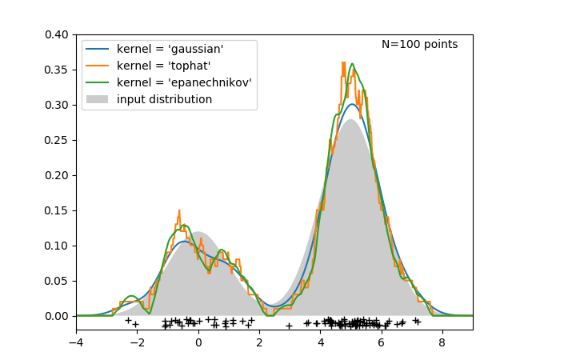如上图，我们利用核密度估计对一个为双峰正态分布的样本进行估计，可以看出，通过核密度估计得到的分布于实际分布还是比较接近，也可以看出不同的核函数，估计出来的分布也是差别的。
核函数
常见的函数主要有以下这些：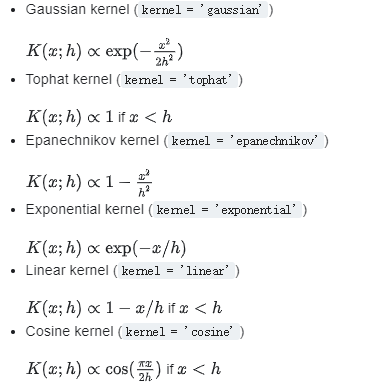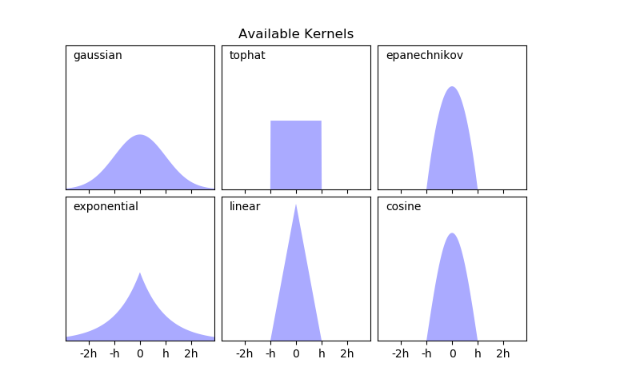python实现
在python中实现核密度估计非常的简单，因为python已经提供了相关的API，直接调用就可以了。
from sklearn.neighbors.kde import KernelDensity
import numpy as np
X = np.array([[-1, -1], [-2, -1], [-3, -2], [1, 1], [2, 1], [3, 2]])
kde = KernelDensity(kernel='gaussian', bandwidth=0.2).fit(X)
kde.score_samples(X)

array([-0.41075698, -0.41075698, -0.41076071, -0.41075698, -0.41075698,
-0.41076071])

其中，最重要就是这三个参数了。bandwidth是上面公式中的h，algorithm就是加速邻近点的搜索算法如KD-Tree等，kernel就是核函数的选择了。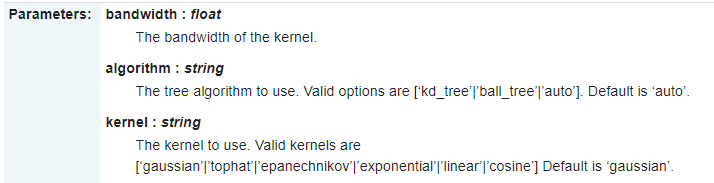Spark实现
其实，spark也提供相应的api。
import org.apache.spark.mllib.stat.KernelDensity

val sample = sc.parallelize(Seq(0.0, 1.0, 4.0, 4.0))
val kd = new KernelDensity()
.setSample(sample)
.setBandwidth(3.0)
val densities = kd.estimate(Array(-1.0, 2.0, 5.0))

但是呢，spark_mllib提供的核密度估计api只支持高斯分布的核函数，最重要的是它只支持一维数据，不支持多维数据的估计
spark多维核密度估计实现
在这里，我自己通过对源码的修改，实现了对多维数据的支持。

首先，先修改setSample方法，让其能够接受多维的数据。

  /**
* Sets the sample to use for density estimation.
*/
def setSample(sample: RDD[Array[Double]]): this.type = {
this.sample = sample
this
}

/**
* Sets the sample to use for density estimation (for Java users).
*/
def setSample(sample: JavaRDD[Array[Double]]): this.type = {
this.sample = sample.rdd.asInstanceOf[RDD[Array[Double]]]
this
}


接下来，就是修改estimate方法，即概率密度值估计的计算方法

  /**
* Estimates probability density function at the given array of points.
*/
def estimate(points: Array[Array[Double]]): Array[Double] = {
val sample = this.sample
val bandwidth = this.bandwidth

require(sample != null, "Must set sample before calling estimate.")

val n = points.length
// This gets used in each Gaussian PDF computation, so compute it up front
val logStandardDeviationPlusHalfLog2Pi = math.log(bandwidth) + 0.5 * math.log(2 * math.Pi)
val (densities, count) = sample.aggregate((new Array[Double](n), 0L))(
// y是对sample的遍历
// x是存放每次返回的Tuple，初始值即为传入的(new Array[Double](n), 0L)
// 每次都将上一轮返回的x作为这一轮的x输入
(x, y) => {
var i = 0
while (i < n) {
var multiply:Double = 1
for (m <- 0 until y.length) {multiply *= normPdf(y(m), bandwidth, logStandardDeviationPlusHalfLog2Pi, points(i)(m))}
x._1(i) += multiply
i += 1
}
(x._1, x._2 + 1)
},
(x, y) => { // 这里是对所有分区的结果进行聚合
blas.daxpy(n, 1.0, y._1, 1, x._1, 1)
(x._1, x._2 + y._2)
})
blas.dscal(n, 1.0 / count, densities, 1)
densities
}
}

normPdf是计算正态分布概率密度值的一个静态类
private object KernelDensity {

/** Evaluates the PDF of a normal distribution. */
def normPdf(
mean: Double,
standardDeviation: Double,
logStandardDeviationPlusHalfLog2Pi: Double,
x: Double): Double = {
val x0 = x - mean
val x1 = x0 / standardDeviation
val logDensity = -0.5 * x1 * x1 - logStandardDeviationPlusHalfLog2Pi
math.exp(logDensity)
}
}

完整代码
完整的代码比较长，我已经上传到GitHub，大家可以去仔细阅读。
欢迎关注同名公众号：“我就算饿死也不做程序员”。
交个朋友，一起交流，一起学习，一起进步。展开全文非参数估计 数据挖掘 python spark
• 前言： {  由于有代码需要调试，这一次也是选择了... 按照维基百科对核密度估计的介绍，核密度估计是一种估计随机变量的概率密度函数的非参数方法，式（1）是公式。 式（1） 其中x是若干数据样本；K()是核...
• 核密度估计参考教案： 根据从一个总体中抽出的样本取估计总体分布的密度函数，在应用上有重要的意义。 关于密度函数的参数估计就是在假定该随即变量的密度函数的形式已知的前提下，对密度函数的参数进行估计。
• ## 核密度估计(Kernel density estimation)

万次阅读 多人点赞 2014-12-15 20:14:28
核密度估计(Kernel density estimation)简析核函数
• matlab核密度估计程序，用于数据的预测，风险控制，预期收益等
• 基于S变换谱核密度估计的齿轮故障诊断.pdf,针对齿轮在故障损伤状态下的振动信号，提出一种基于S变换谱二维核密度估计的冲击特征提取方法，以实现齿轮的故障诊断。该方法首先对包含冲击特征的振动信号进行S变换；然后...
• 主要用MATLAB编写的核密度估计方法，采用的核是高斯核！
• 论文研究-基于变换核密度估计的半参数GARCH模型.pdf, 针对金融资产收益率分布呈现的尖峰、厚尾及有偏的特点，沿袭变换核密度估计的思想，提出一种广义Logistic变换，对...
• 东华大学机器学习核密度估计大作业 第一部分是一个三维的彩色KDE估计图（最好用MATLAB画）；第二部分是测试图片的运动目标二值图像检测结果（运动员用白色像素，背景用黑色）
• 针对密度峰值聚类算法CFSFDP（clustering by fast search and find of density peaks）计算密度时人为判断截断距离和人工截取簇类中心的缺陷，提出了一种基于非参数核密度估计的密度峰值聚类算法。首先，应用非参数...聚类
• 这个资源是matlab实现的基于KDE（核密度估计）的行人检测，包含代码和数据·库。清清楚楚，明明白白，注释易懂。本人课堂大作业的完整版。
• MATLAB实现的二维核密度估计。 输入平面样本点，得到概率密度函数。 2D Kernel Density Estimation。2D KDE
• kde核密度估计，非参数估计的一种，使用高斯核函数进行概率密度估计，应用于独立成分分析确定控制限等过程
• 东华大学核密度估计KDE代码 第一部分是一个三维的彩色KDE估计图（最好用MATLAB画）；第二部分是测试图片的运动目标二值图像检测结果（运动员用白色像素，背景用黑色）
• ## 核密度估计简介

千次阅读 2013-04-03 16:36:40
核密度估计又叫核函数估计。 kernel density estimation是在概率论中用来估计未知的密度函数，属于非参数检验方法之一，由Rosenblatt (1955)和Emanuel Parzen(1962)提出，又名Parzen窗（Parzen window）。Ruppert和...
• ## 核密度估计KDE

千次阅读 2018-08-19 11:28:49
核密度估计Kernel Density Estimation(KDE)概述 密度估计的问题 由给定样本集合求解随机变量的分布密度函数问题是概率统计学的基本问题之一。解决这一问题的方法包括参数估计和非参数估计。 参数估计 参数估计又...
• 多元核密度估计 承接上一篇文章,接着讨论多维随机变量情况下的核密度估计。 定义 给定一组样本X={x1,x2,⋯ ,xn}\bm{X}=\{ \bm{x}_1,\bm{x}_2,\cdots,\bm{x}_n \}X={x1​,x2​,⋯,xn​}且都是ddd维的向量,并且取自同......